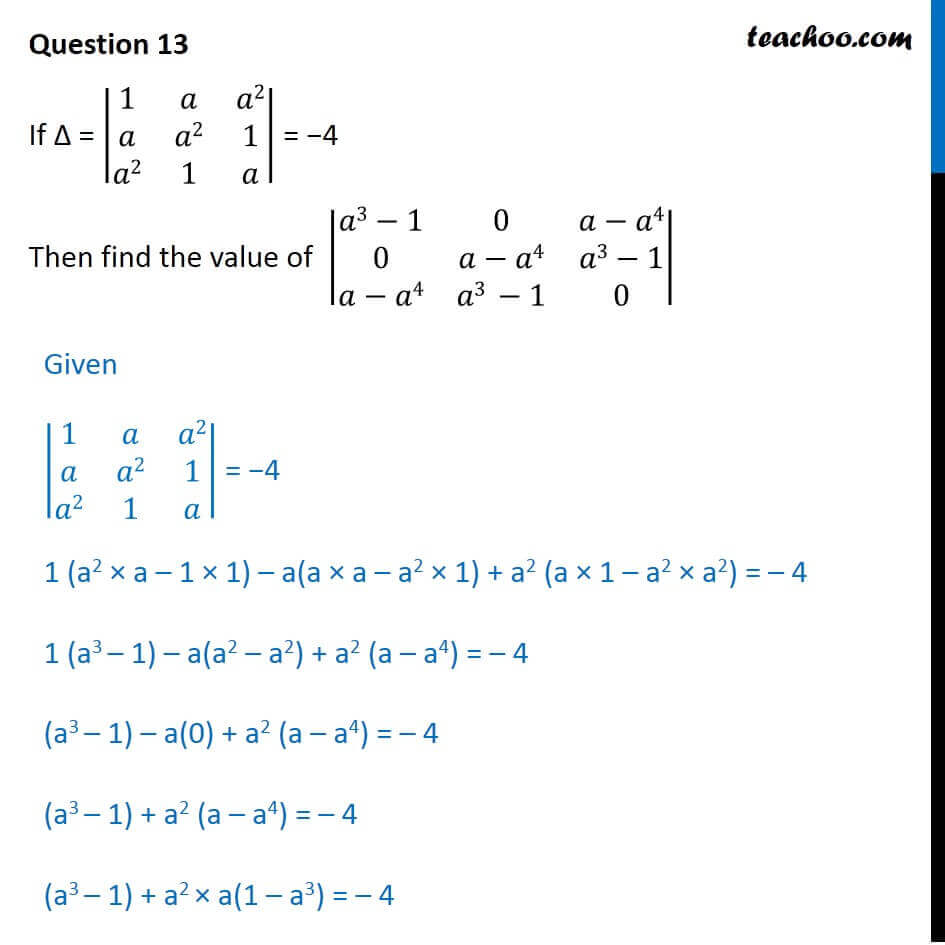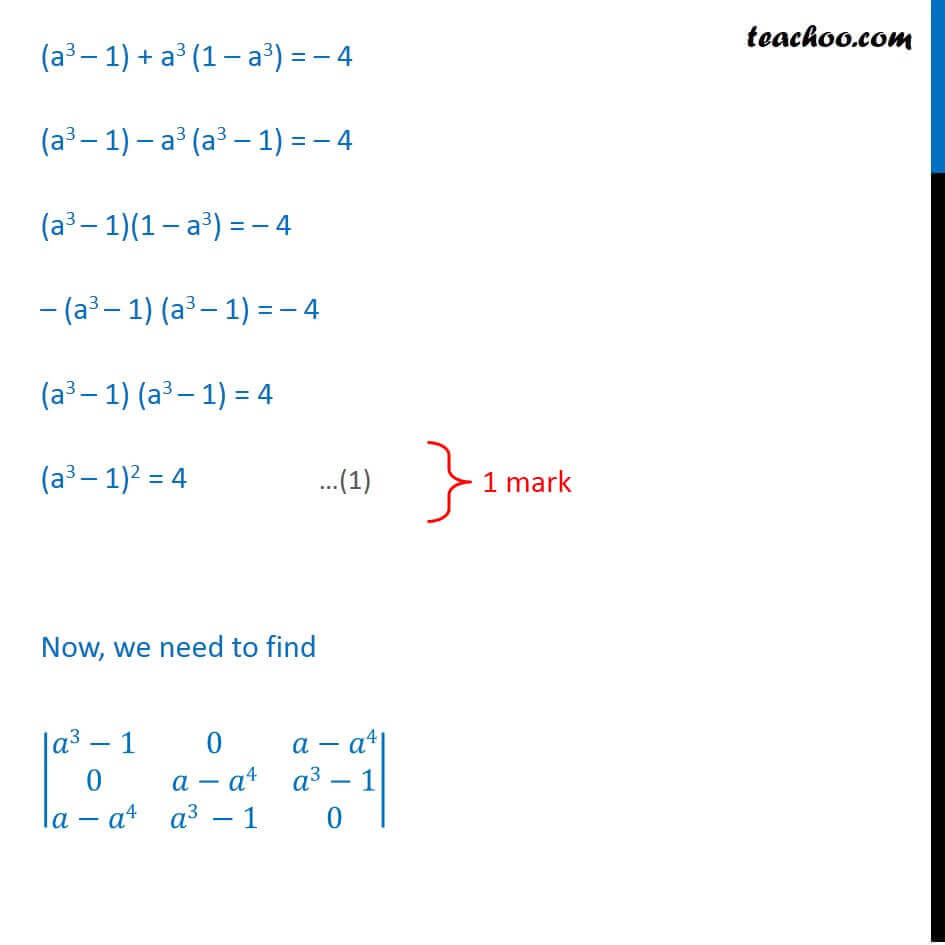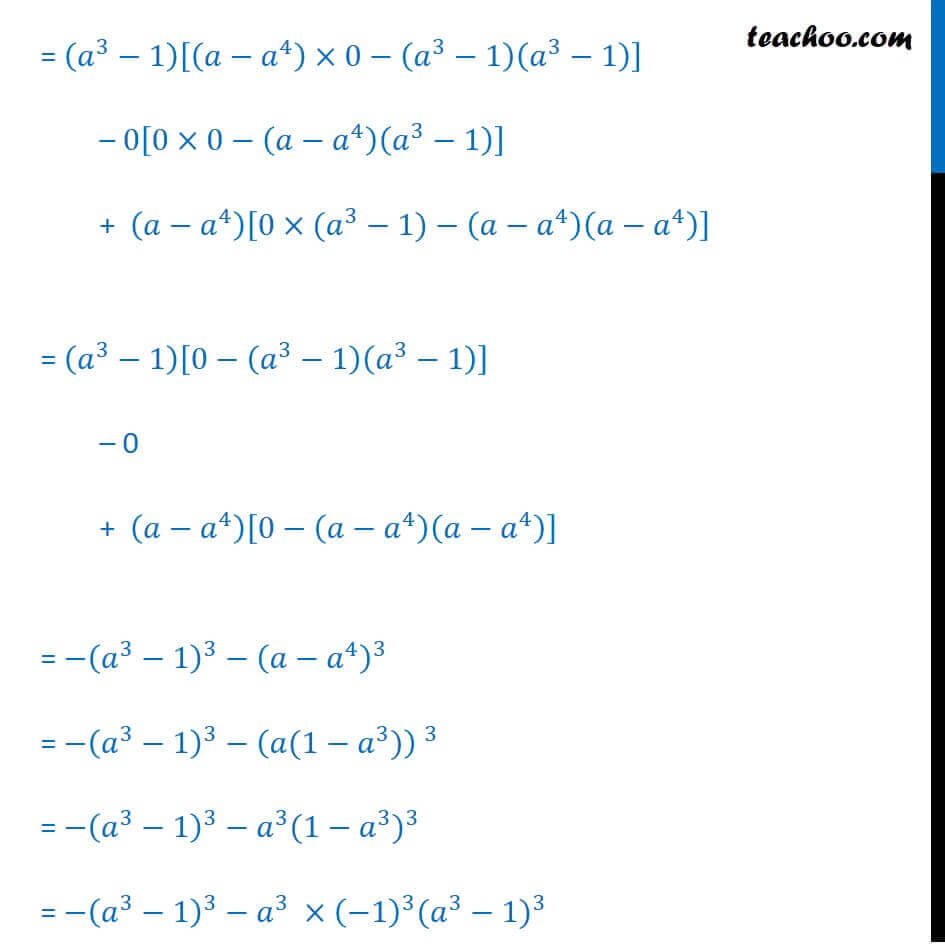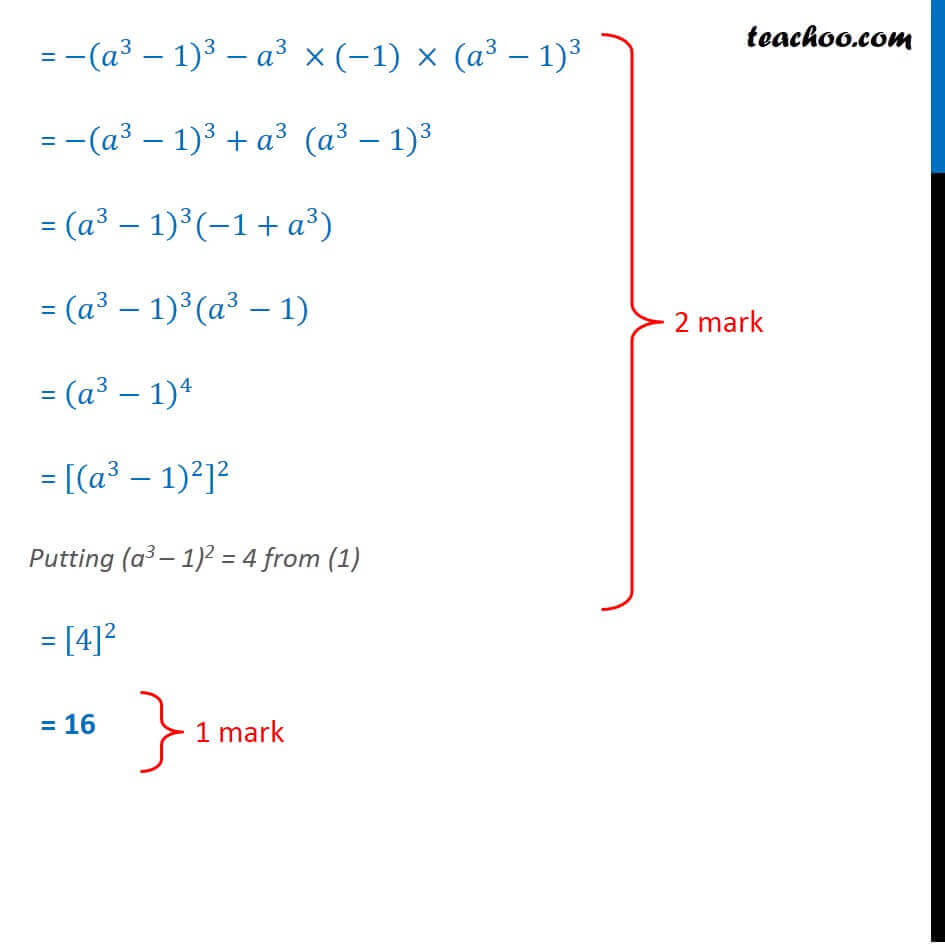CBSE Class 12 Sample Paper for 2018 Boards

Class 12
Solutions of Sample Papers and Past Year Papers - for Class 12 Boards

This is a question of CBSE Sample Paper - Class 12 - 2017/18.Learn in your speed, with individual attention - Teachoo Maths 1-on-1 Class

### Transcript

Question 13 If ∆ = |■8(1&𝑎&𝑎2@𝑎&𝑎2&1@𝑎2&1&𝑎)| = −4 Then find the value of |■8(𝑎3−1&0&𝑎−𝑎4@0&𝑎−𝑎4&𝑎3−1@𝑎−𝑎4&𝑎3 −1&0)| Given |■8(1&𝑎&𝑎2@𝑎&𝑎2&1@𝑎2&1&𝑎)| = −4 1 (a2 × a – 1 × 1) – a(a × a – a2 × 1) + a2 (a × 1 – a2 × a2) = – 4 1 (a3 – 1) – a(a2 – a2) + a2 (a – a4) = – 4 (a3 – 1) – a(0) + a2 (a – a4) = – 4 (a3 – 1) + a2 (a – a4) = – 4 (a3 – 1) + a2 × a(1 – a3) = – 4 (a3 – 1) + a3 (1 – a3) = – 4 (a3 – 1) – a3 (a3 – 1) = – 4 (a3 – 1)(1 – a3) = – 4 – (a3 – 1) (a3 – 1) = – 4 (a3 – 1) (a3 – 1) = 4 (a3 – 1)2 = 4 Now, we need to find |■8(𝑎3−1&0&𝑎−𝑎4@0&𝑎−𝑎4&𝑎3−1@𝑎−𝑎4&𝑎3 −1&0)| = (𝑎^3−1)[(𝑎−𝑎^4 )×0−(𝑎^3−1)(𝑎^3−1)] – 0[0×0−(𝑎−𝑎^4 )(𝑎^3−1)] + (𝑎−𝑎^4 )[0×(𝑎^3−1)−(𝑎−𝑎^4 )(𝑎−𝑎^4 )] = (𝑎^3−1)[0−(𝑎^3−1)(𝑎^3−1)] – 0 + (𝑎−𝑎^4 )[0−(𝑎−𝑎^4 )(𝑎−𝑎^4 )] = −(𝑎^3−1)^3−(𝑎−𝑎^4 )^3 = −(𝑎^3−1)^3−〖(𝑎(1−𝑎^3)) 〗^3 = −(𝑎^3−1)^3−〖𝑎^3 (1−𝑎^3)〗^3 = −(𝑎^3−1)^3−〖𝑎^3 ×(−1)^3 (𝑎^3−1)〗^3 = −(𝑎^3−1)^3−〖𝑎^3 ×(−1)^3 (𝑎^3−1)〗^3 = −(𝑎^3−1)^3−〖𝑎^3 ×(−1) × (𝑎^3−1)〗^3 = −(𝑎^3−1)^3+〖𝑎^3 (𝑎^3−1)〗^3 = (𝑎^3−1)^3 (−1+𝑎^3) = (𝑎^3−1)^3 (𝑎^3−1) = (𝑎^3−1)^4 = [(𝑎^3−1)^2 ]^2 Putting (a3 – 1)2 = 4 from (1) = ^2 = 16 = −(𝑎^3−1)^3−〖𝑎^3 ×(−1)^3 (𝑎^3−1)〗^3 = −(𝑎^3−1)^3−〖𝑎^3 ×(−1) × (𝑎^3−1)〗^3 = −(𝑎^3−1)^3+〖𝑎^3 (𝑎^3−1)〗^3 = (𝑎^3−1)^3 (−1+𝑎^3) = (𝑎^3−1)^3 (𝑎^3−1) = (𝑎^3−1)^4 = [(𝑎^3−1)^2 ]^2 Putting (a3 – 1)2 = 4 from (1) = ^2 = 16